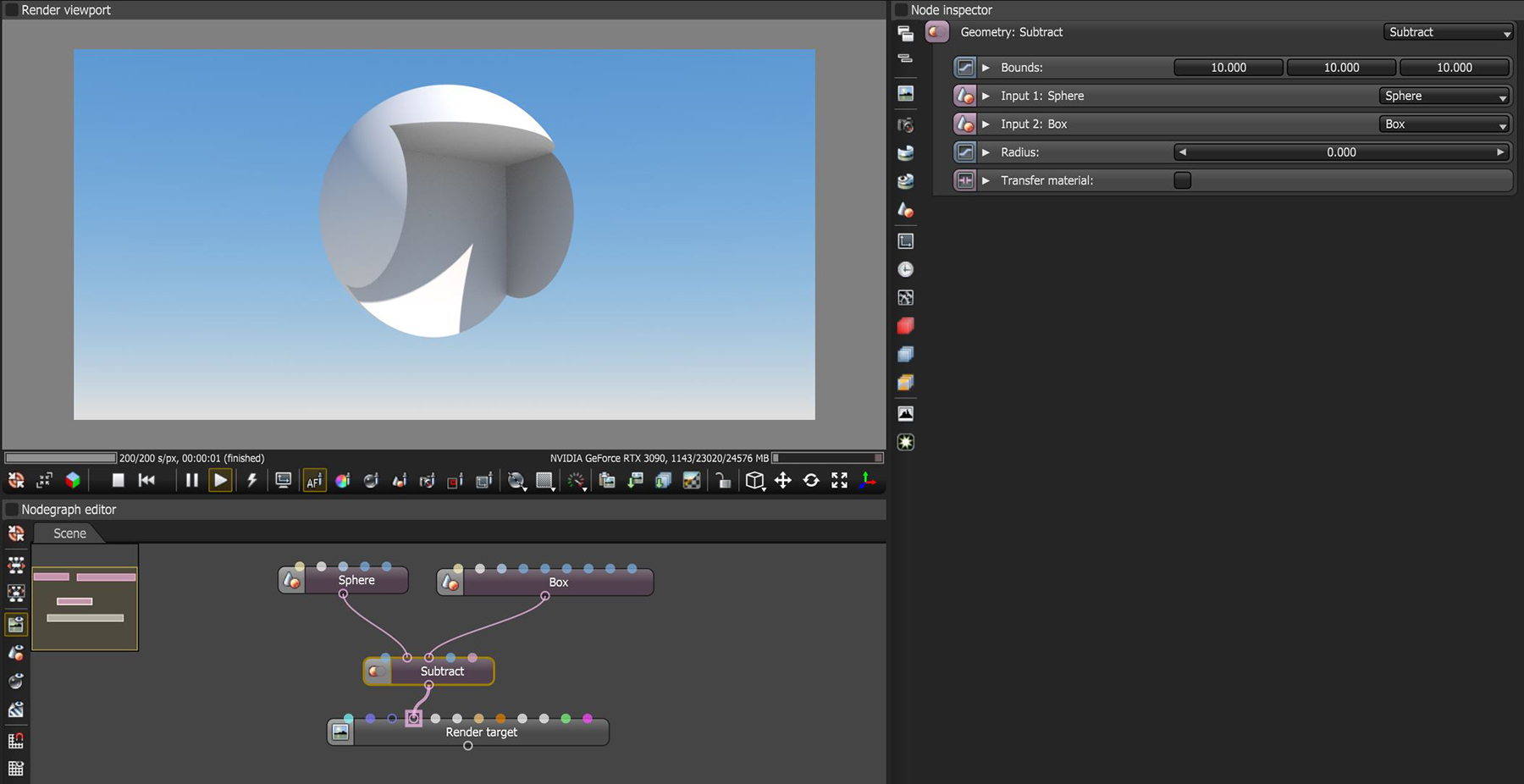# Subtract

The Subtract node will output the subtraction of the second input from the first (figure 1).Figure 1: A Vectron cube being subtracted from a Vectron sphere

## Subtract Parameters

Bounds - Determines the bounds of the Vectron geometry.

Input 1 - The base Vectron object.

Input 2 - The Vectron object that will subtracted from Input 1.

Radius - Increases the intersection area, higher values will produce smoother results.

Transfer MaterialThe representation of the surface or volume properties of an object. - If enabled, the material on the second input will be kept on the intersecting results.